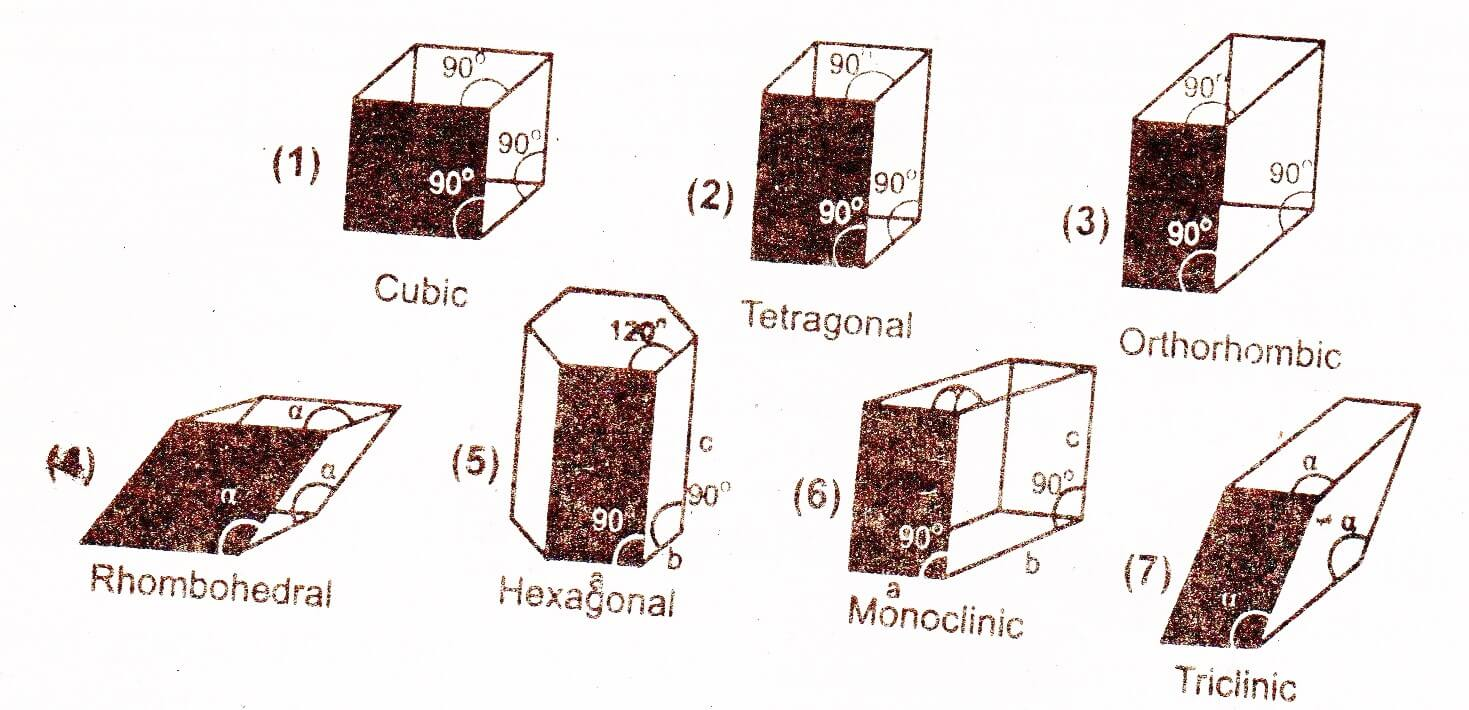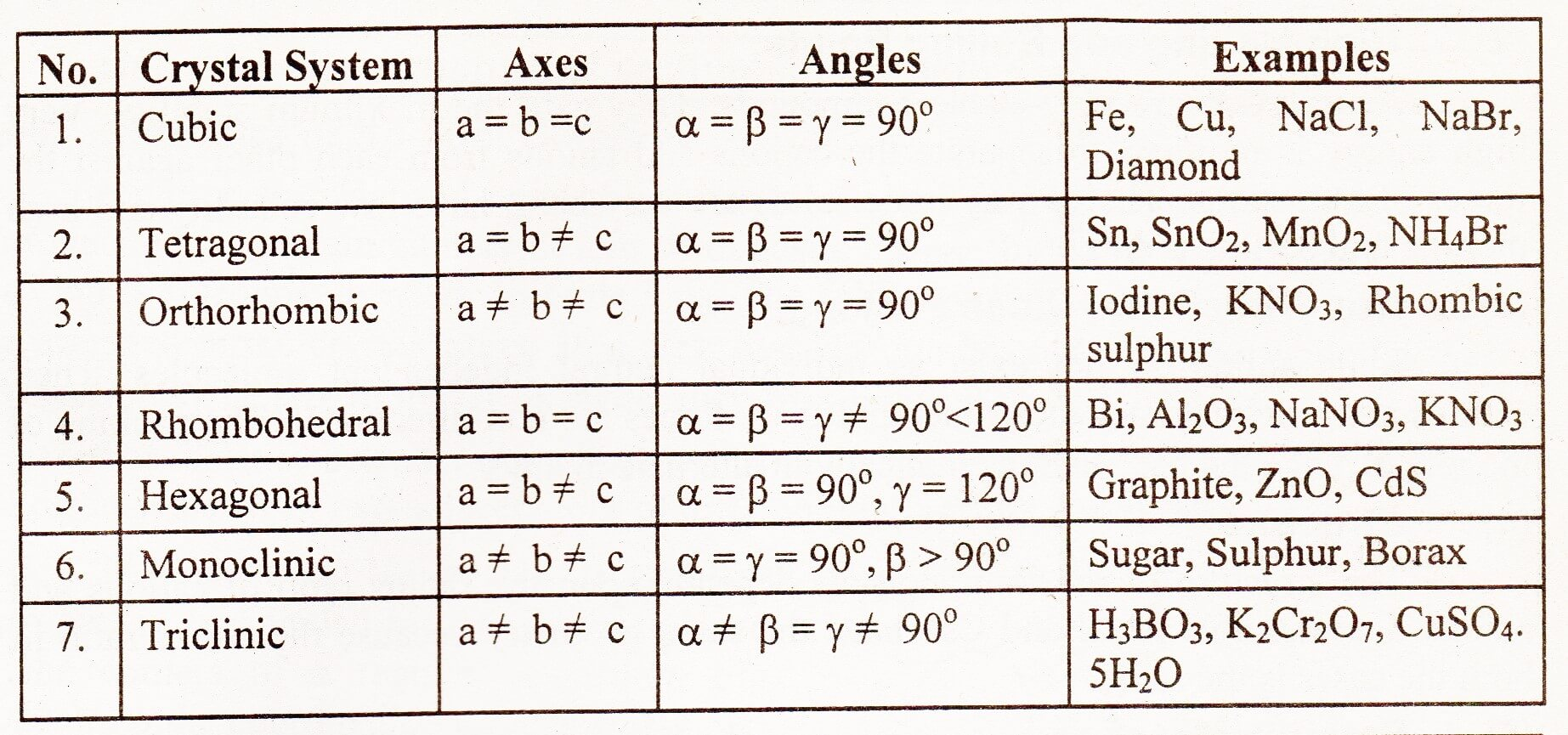In 1850, a French mathematician August Bravis observed that crystal lattice of substances can be categorized into seven types. These are called Bravis Lattices and their units cells are referred to as Bravis unit cells. A crystal system may be identified by the dimensions of a unit cell along its three edges or axe, a, b, c and three angles between the axes α, β, γ. These seven crystal systems are described as follows:1. ## Cubic system

In this system all the three axes are of equal lengths and all are at right angle to one another.

1. ## Tetragonal system

In this system two axes are of equal lengths and the third axis is either shorter or larger than the other two. All angles are 90°.

1. ## Orthorhombic or Rhombic system

All the three axes are of unequal length and all are at right angle to each another.

1. ## Rhombohedral system Or Trigonal system

All the three axes are of an equal length like cubic system but the three angles are not equal and lie between 90° and 120°.

1. ## Hexagonal system

In this system two axes are of equal length and are in one plane making an angle of 120° with each other. The third axis which is different in length than the other two is at right angle to these two axes.

1. ## Monoclinic system

All the three axes are of unequal length; two of these axes are at right angle to each other while the third angle is greater than 90°.

1. ## Triclinic system

All the three axes and the three angles are unequal and none of the angles is 90°.